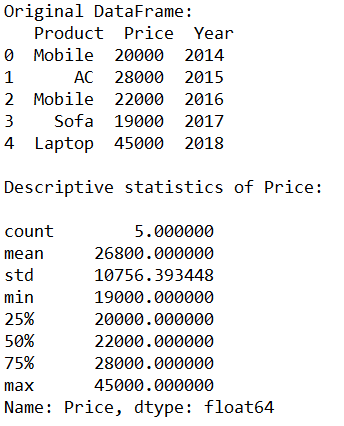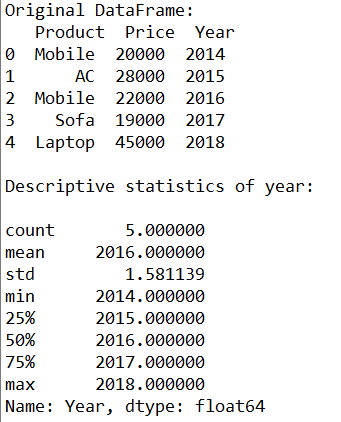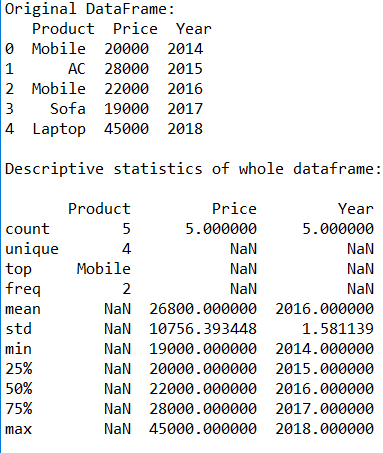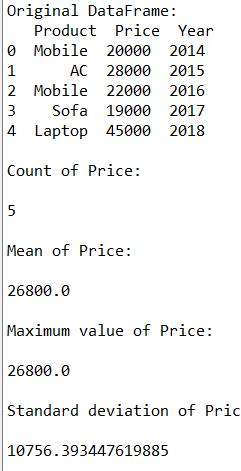Related Articles
How to Get the Descriptive Statistics for Pandas DataFrame?
• Difficulty Level : Basic
• Last Updated : 10 Jul, 2020

`describe()` method in Python Pandas is used to compute descriptive statistical data like count, unique values, mean, standard deviation, minimum and maximum value and many more. In this article, let’s learn to get the descriptive statistics for Pandas DataFrame.

Syntax:
df[‘cname’].describe(percentiles = None, include = None, exclude = None)
df.describe(percentiles = None, include = None, exclude = None)

Parameters:
percentiles: represents percentile value that has to be returned by the function. Default values are 0.25, 0.5 and 0.75
include: represents list of data types that has to be included
exclude: represents list of data types that has to be excluded

Example 1:

 `# Import package``from` `pandas ``import` `DataFrame`` ` `# Create DataFrame``cart ``=` `{``'Product'``: [``'Mobile'``, ``'AC'``, ``'Mobile'``, ``'Sofa'``, ``'Laptop'``],``        ``'Price'``: [``20000``, ``28000``, ``22000``, ``19000``, ``45000``],``         ``'Year'``: [``2014``, ``2015``, ``2016``, ``2017``, ``2018``]``        ``}``df ``=` `DataFrame(cart, columns ``=` `[``'Product'``, ``'Price'``, ``'Year'``])`` ` `# Original DataFrame``print``(``"Original DataFrame:\n"``, df)`` ` `# Describing descriptive statistics of Price``print``(``"\nDescriptive statistics of Price:\n"``)``stats ``=` `df[``'Price'``].describe()``print``(stats)`

Output:Example 2:

 `# Import package``from` `pandas ``import` `DataFrame`` ` `# Create DataFrame``cart ``=` `{``'Product'``: [``'Mobile'``, ``'AC'``, ``'Mobile'``, ``'Sofa'``, ``'Laptop'``],``        ``'Price'``: [``20000``, ``28000``, ``22000``, ``19000``, ``45000``],``         ``'Year'``: [``2014``, ``2015``, ``2016``, ``2017``, ``2018``]``        ``}``df ``=` `DataFrame(cart, columns ``=` `[``'Product'``, ``'Price'``, ``'Year'``])`` ` `# Original DataFrame``print``(``"Original DataFrame:\n"``, df)`` ` `# Describing descriptive statistics of Year``print``(``"\nDescriptive statistics of year:\n"``)``stats ``=` `df[``'Year'``].describe()``print``(stats)`

Output:Example 3:

 `# Import package``from` `pandas ``import` `DataFrame`` ` `# Create DataFrame``cart ``=` `{``'Product'``: [``'Mobile'``, ``'AC'``, ``'Mobile'``, ``'Sofa'``, ``'Laptop'``],``        ``'Price'``: [``20000``, ``28000``, ``22000``, ``19000``, ``45000``],``         ``'Year'``: [``2014``, ``2015``, ``2016``, ``2017``, ``2018``]``        ``}``df ``=` `DataFrame(cart, columns ``=` `[``'Product'``, ``'Price'``, ``'Year'``])`` ` `# Original DataFrame``print``(``"Original DataFrame:\n"``, df)`` ` `# Describing descriptive statistics of whole dataframe``print``(``"\nDescriptive statistics of whole dataframe:\n"``)``stats ``=` `df.describe(include ``=` `'all'``)``print``(stats)`

Output:Example 4:
In this example, let’s print all the descriptive statistical data individually.

 `from` `pandas ``import` `DataFrame`` ` `# Create DataFrame``cart ``=` `{``'Product'``: [``'Mobile'``, ``'AC'``, ``'Mobile'``, ``'Sofa'``, ``'Laptop'``],``        ``'Price'``: [``20000``, ``28000``, ``22000``, ``19000``, ``45000``],``         ``'Year'``: [``2014``, ``2015``, ``2016``, ``2017``, ``2018``]``        ``}``df ``=` `DataFrame(cart, columns ``=` `[``'Product'``, ``'Price'``, ``'Year'``])`` ` `# Original DataFrame``print``(``"Original DataFrame:\n"``, df)`` ` `# Print Count of Price``print``(``"\nCount of Price:\n"``)``counts ``=` `df[``'Price'``].count()``print``(counts)`` ` `# Print mean of Price``print``(``"\nMean of Price:\n"``)``m ``=` `df[``'Price'``].mean()``print``(m)`` ` `# Print maximum value of Price``print``(``"\nMaximum value of Price:\n"``)``mx ``=` `df[``'Price'``].``max``()``print``(m)`` ` `# Print standard deviation of Price``print``(``"\nStandard deviation of Price:\n"``)``sd ``=` `df[``'Price'``].std()``print``(sd)`

Output:Attention geek! Strengthen your foundations with the Python Programming Foundation Course and learn the basics.

To begin with, your interview preparations Enhance your Data Structures concepts with the Python DS Course. And to begin with your Machine Learning Journey, join the Machine Learning – Basic Level Course

My Personal Notes arrow_drop_up